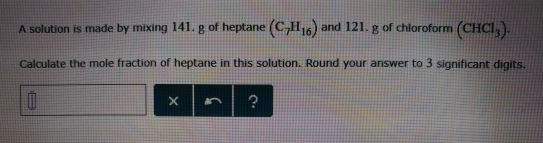# Problem: A solution is made by mixing 141. g of heptane (C7H16) and 121. g of chloroform (CHCl3) Calculate the mole fraction of heptane in this solution. Round your answer to 3 significant digits.

###### FREE Expert Solution
84% (117 ratings)###### Problem Details

A solution is made by mixing 141. g of heptane (C7H16) and 121. g of chloroform (CHCl3) Calculate the mole fraction of heptane in this solution. Round your answer to 3 significant digits.